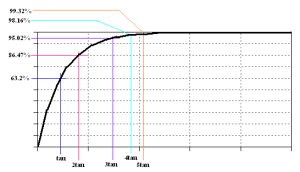# Charging and Discharging Capacitive Circuits Detailed Explanation

## Charging and Discharging Capacitive Circuits

The voltage on a circuit having capacitors will not immediately go to its settling state unlike purely resistive circuits. When a potential difference is applied to an RC circuit the like of Figure 31 below and then S1 is closed, the voltage across the capacitor will exponentially rises from zero to its final value. At this condition as well, the current is exponentially decaying with a peak value at zero potential across the capacitor until it reach to its dc state when the capacitor is fully charged. The moment in which the voltage and the current of the capacitor are changing is called a transient condition. Transient condition that is happening during startup is due to the charging of the capacitor present in the circuit.

The peak current due to capacitor transient is very high that design engineers should not take for granted for this may damage the other components in the circuit. The transient current of Figure 31 when S1 is closed is proportional to the product of the capacitance and the rate of change of the voltage as below.This is the current magnitude that will flow to resistor R and capacitor C at the moment S1 is closed. This current is not permanent (as described by the term transient) and will be settle to zero once there is no more change in the applied voltage. Once the settling stage is reached, the current in the circuit is dictated by the resistance of resistor R and the load of the circuit.

## Charging the Capacitor

The capacitor will start to charge when S1 is closed while S2 remains open as Figure 32. At this instance, the sum of the current in the resistor and the capacitor is always equal to zero. This is due to the 180 degrees phase difference between the two currents. If we define the resultant current algebraically, it will beRe-arranging terms will result toIf you recall your differential equations, the solution for this isThis is the voltage seen in the output with respect to time which is also equal to the voltage across the capacitor C of Figure 32.

Therefore, the voltage across C is can be rewritten asThe voltage across the resistor is equal to the input voltage V minus the voltage of the capacitor C. Mathematically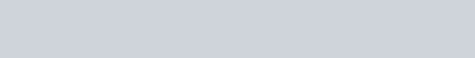By using LTSpice simulation software (click here for LTspice step by step tutorials), we can view the respective waveform of R and C as depicted in Figure 33. The waveform going upward (green) is the voltage of the capacitor while the going downward (blue) is the waveform for the resistor voltage. In this simulation the supply voltage is 10V and a particular value for R and C. You can see here that at first the voltage of the capacitor is zero then exponentially settling to its input voltage (at this time the capacitor is fully charged). You can simulate this behavior using any spice based software.

## Time Constant

The settling or the final value of the voltage across the capacitor C in Figure 32 is attainable during 5 time constants. One time constant or commonly known as the tau is equal to the product of resistance R and capacitance C. Mathematically,Time constant tau is in seconds while R and C are ohms and farad respectively. At first time constant the charge on the capacitor as defined by [Eq. 37] will be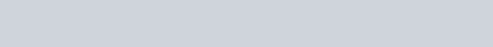Therefore the charge of C at one time constant is equal to 63.2% of the input voltage V. By using same equation, the amount of charge present at 5 time constants will be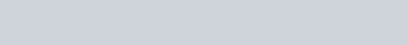At 5 time constants, the charge of the capacitor is already 99.32% which means that it is entering the steady state condition. Figure 34 shows the corresponding potential present in the capacitor for every time constant.

### Example 7

Considering the circuit in Figure 35,

a.) How long will it take to reach the first time constant?

b.) What is the settling time of the circuit?

c.) What is the rise time of the circuit?

d.) What is the peak current experienced by R?

e.) What will be the voltage of R at 5 time constants?

f.) What will be the current in C at 10msec?

g.) What should be the value of C to attain final level by 20msec? (Consider same resistance)

### Solution

a) tau=RC=(1k) (10u) = 10msec

b) The circuit will attain its steady state at five time constants, therefore the settling time is

5tau = (5) (10msec) = 50msec

c) Actually the maximum rise time of a circuit is equal to its settling time. However if we take account the accepted standard in analog electronics, the rise time should be measured from 10% to 90% of the steady state level of the rising waveform.

At 10% of the rising waveform, the level of the output is already 10% of the input voltage. (When the capacitor is fully charged, the level seen in the output is equal to the input since the capacitive reactance during this time is very high which approaches open circuit.) So by using [Eq. 37] we can get the time equivalent to 10% of V asNow we’re going to solve the time it takes to reach 90% of the final value.The rise time of the circuit will be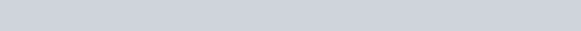d) The peak current experienced by R is happening when capacitor C is zero charge which takes place ideally at time zero. When the capacitor voltage is zero, the voltage across the resistor R is maximum, thus,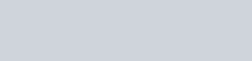e) The voltage of R at 5 time constants will be

## Discharging a Capacitor

Transients are not only happening during start up but also during turn off. When a fully charged capacitor is connected across a load and the supply is removed, its charge will be transferred to the load and there happening transient condition as well. When S1 in Figure 36 is closed, capacitor C is charging to full load. When S1 is suddenly opened at the same time S2 is closed the charge on capacitor C will be discharged to resistor R. The discharge follows exponential fashion as with [Eq. 38].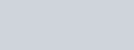(Where  is the initial voltage of the capacitor)### Example 8

The full charge voltage of the capacitor in Figure 36 is 10V. What will be the voltage measured on R when S1 is open while S2 is closed at the same time after 20msec? The value of R and C are 100Ω and 100µF respectively.

### SolutionIn actual circuit design, it is very important to understand charging and discharging capacitive circuits to avoid component damage due to high peak currents. To easily understand, I recommend that you will use a simulation software to view the behavior of the circuit. There are plenty of free circuit simulators that you can use such as LTspice. Click here for step by step circuit simulation tutorials.

This site uses Akismet to reduce spam. Learn how your comment data is processed.